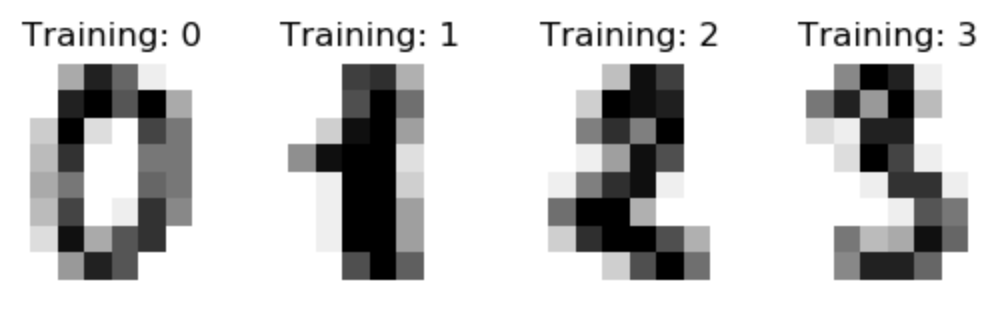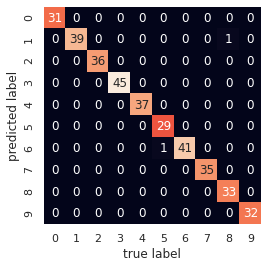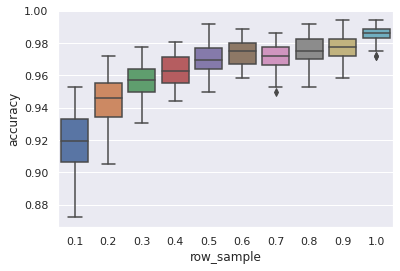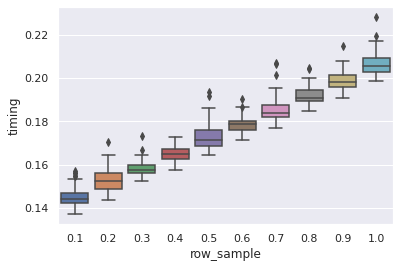`AdaOpt` is a probabilistic classifier based on a mix of multivariable optimization and a nearest neighbors algorithm. More details about it are found in this paper. When reading the paper, keep in mind that the algorithm is still very new; only time will allow to fully appreciate all of its features. Plus, its performance on this dataset is not an indicator of its future performance, on other datasets.

Currently, the package containing `AdaOpt`, `mlsauce`, can be installed from the command line as:

``````pip install git+https://github.com/thierrymoudiki/mlsauce.git

``````

In this post, we’ll use `mlsauce`’s `AdaOpt` on a handwritten digits dataset from UCI Machine Learning repository.The model is firstly trained on a set of digits – to distinguish between a “3”, or a”6”, etc.:

``````from time import time
from tqdm import tqdm
import mlsauce as ms
import numpy as np
from sklearn.metrics import classification_report
from sklearn.model_selection import train_test_split

Z = digits.data
t = digits.target

# Split data in training and testing sets
np.random.seed(2395)
X_train, X_test, y_train, y_test = train_test_split(Z, t,
test_size=0.2)

learning_rate=0.3,
reg_lambda=0.1,
reg_alpha=0.5,
eta=0.01,
gamma=0.01,
tolerance=1e-4,
row_sample=1,
k=3)

# Teaching AdaOpt to recognize digits
start = time()
obj.fit(X_train, y_train)
print(time()-start)

``````
``````0.03549695014953613
``````

Then, `AdaOpt` is tasked to recognize new, unseen digits `(X_test, y_test)`, based on what it has seen on the training set `(X_train, y_train)`:

``````start = time()
print(obj.score(X_test, y_test))
print(time()-start)
``````
``````0.9944444444444445
0.19525575637817383
``````

The accuracy is high on this dataset. Additional error metrics are presented in the following table:

``````preds = obj.predict(X_test)
print(classification_report(preds, y_test))

``````
``````              precision    recall  f1-score   support

0       1.00      1.00      1.00        31
1       1.00      0.97      0.99        40
2       1.00      1.00      1.00        36
3       1.00      1.00      1.00        45
4       1.00      1.00      1.00        37
5       0.97      1.00      0.98        29
6       1.00      0.98      0.99        42
7       1.00      1.00      1.00        35
8       0.97      1.00      0.99        33
9       1.00      1.00      1.00        32

accuracy                           0.99       360
macro avg       0.99      1.00      0.99       360
weighted avg       0.99      0.99      0.99       360

``````

Ad here is a confusion matrix:At test time, `AdaOpt` uses a nearest neighbors algorithm. Which means, a task with quadratic complexity (a large number of operations). But there are a few tricks implemented in `mlsauce`’s `AdaOpt` to alleviate the potential burden on very large datasets, such as: instead of comparing the testing set to the whole training set, comparing it to a stratified subsample of the training set.

`row_sample == 0.1` for example in the next figure, means that 1/10 of the training set is used in the nearest neighbors procedure at test time. The figure represents a distribution of test set accuracy:We also have the following timings in seconds (current, could be faster in the future) for training+prediction, as a function of `row_sample`:The paper contains a more detailed discussion of how these figures are obtained, and a description of `AdaOpt`.

Note: I am currently looking for a gig. You can hire me on Malt or send me an email: thierry dot moudiki at pm dot me. I can do descriptive statistics, data preparation, feature engineering, model calibration, training and validation, and model outputs’ interpretation. I am fluent in Python, R, SQL, Microsoft Excel, Visual Basic (among others) and French. My résumé? Here!